## How do I increase student interactivity when using PhET simulations in lecture?

posted June 28, 2016 and revised November 17, 2022
by Stephanie Chasteen and Yuen-ying Carpenter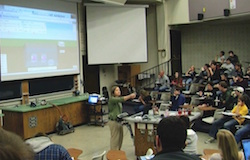PhET simulations are free, online interactive simulations for teaching and learning science. The simulations emphasize visual models, cause-and-effect relationships, and multiple representations. Click here to see all Expert Recommendations on the use of PhET.

While the simulations are effective when used as a lecture demonstration, the impact of these demonstrations is greatly increased when students are given the opportunity to interact with the simulations. While students can interact with the simulations on their own computers in a class or lab environment, there are also several ways to encourage inquiry when the teacher is the one controlling the simulation.

Short video on using PhET interactively in a lecture setting.

### How do I facilitate a class discussion around PhET simulations?

PhET simulations allows you to change variables and discover cause-and-effect relationships, promoting the development of scientific inquiry skills. Even in a classroom where the instructor is the only person controlling the simulation, some of the power of this scientific inquiry can be captured during whole-class discussions (Moore et al. 2014). We call this kind of discussion "whole class inquiry," because the whole class can engage with testing cause-and-effect relationships as if they were one large lab group.

#### How does this actually work?

1. Demonstrate one experiment or scenario in the simulation. It may help to highlight the variables and settings that you’ve chosen, to give students ideas about what controls are available.
2. Ask students questions based on this demonstration. For example, what will happen if I turn on friction? What affects the period of a pendulum? Then use the simulation to leverage the features of the simulation to guide the discussion.
3. Encourage students to propose new questions and experiments. If it’s still the first few times students have been asked to suggest ideas, give them starter prompts to help, like “What if…?” or “Will it be higher (or lower) if we…” or “What should we change in order to….?” The more often you use the simulations, the more students will become comfortable asking spontaneous questions.
4. Ask for other students’ predictions in response to these suggestions and questions.
5. Discuss and evaluate the reasoning behind any predictions. Even when students agree on the predicted outcome, they may have differing reasons that should be compared, and perhaps followed-up with further tests to see if these reasons always hold.
6. Test the students’ proposal(s) with the simulation to see if the outcome matches their predictions.

#### What are some examples?Buoyancy simulation; the large block is neutrally buoyant.
1. Using the Buoyancy simulation, an instructor might ask students "What should I do to make this neutrally-buoyant block float?" Students can discuss with their neighbors, and propose different scenarios for the instructor to test.
2. With Energy Skate Park Basics, you might start by showing a skater riding on a frictionless track. Suppose a student asks: “What will happen if you add friction?” Instead of giving them the answer immediately, ask the class a follow-up question that directs students to think about the key ideas, e.g. “What will happen to the total energy if friction is included?” Solicit any conflicting student ideas here -- will total energy go down because energy is lost to heat, or is total energy still conserved? Use the simulation and the bar-chart representation of energy to show students the results and resolve this conflict. Students can see that the thermal energy increases, while the kinetic energy decreases, resulting in a constant total energy.

### How do I pair PhET simulations with Peer Instruction and Clicker Questions?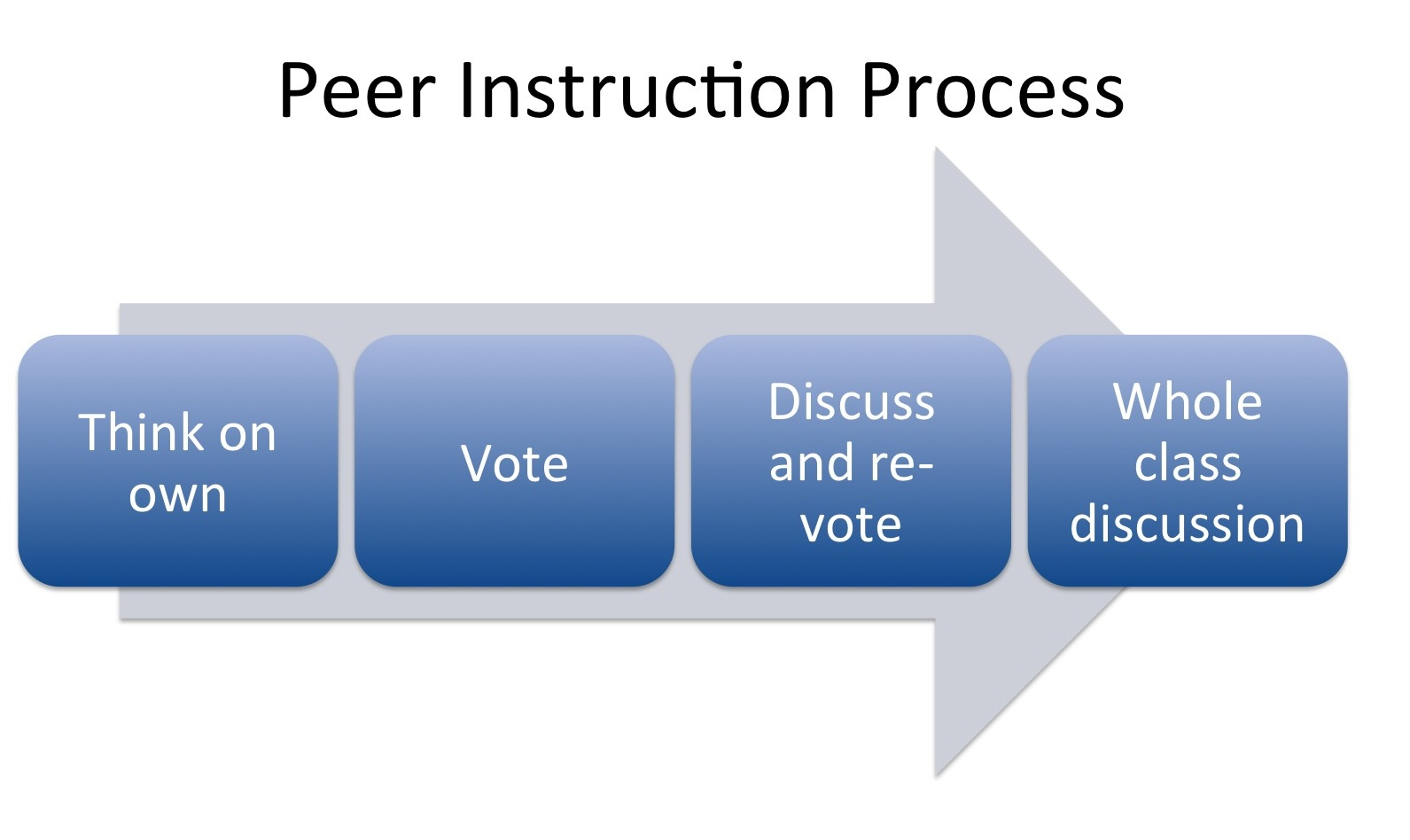Another way to ask students to think about what is happening in the simulation is through clicker questions and peer instruction (Keller et al. 2006; Moore, Herzog, and Perkins 2013; Moore et al. 2014). Clicker questions are multiple-choice questions, designed to give students an opportunity to discuss and make sense of concepts related to the simulation. Students think about the question on their own, and vote, and then discuss with their neighbors to explain and understand each others' reasoning. After a final vote, the instructor holds a whole-class discussion about the question and the reasons behind the answers. Recommendations for facilitating the Peer Instruction in the classroom can be found here.

#### What are some techniques for writing effective clicker questions with PhET?

In addition to general tips for writing good questions for use with clickers and Peer Instruction, questions based on PhET can be made more powerful by using the following techniques:

• Use images from the simulation. In the question, the answer choices, and/or a slide following the question that demonstrates the answer, use images from the simulation to make use of the visual model that students are already familiar with from the simulation.
• Use the simulation to verify the answer. PhET simulations can be seen as virtual experiments – rather than telling students the answer to the clicker question, show them the answer – by closing the switch, testing the voltage, or displaying the graph.
• Generate further inquiry. Because PhET simulations are very flexible, you can use the simulation to generate further open-ended questioning. What will happen if I add a second bulb to the circuit? What if I move the zero-voltage line? This can deepen and further the discussion.

Clicker questions for use with each simulation can be found on our website -- either look on each simulation page, under For Teachers, or browse all clicker questions at Browse Activities.

In general, clicker questions should be stimulating and challenging, rather than simply asking students to recall something that has already been explained. Below are several types of questions that have been successful when paired with PhET simulations:  Prediction, open-ended prediction, contrasting cases, and interpreting representations.

#### Prediction questions

Because PhET simulations are designed to help students explore cause-and-effect relationships, it can be useful to ask students to predict what will happen when a variable or parameter is changed. Then you can use the simulation as an experiment to test their prediction.

For example, in the “Wave on a String” simulation, ask students to apply what they have learned about waves reflecting at a boundary to predict behavior in a new situation. You can follow up by inverting the wave, removing the fixed end, or asking students what they would like to try.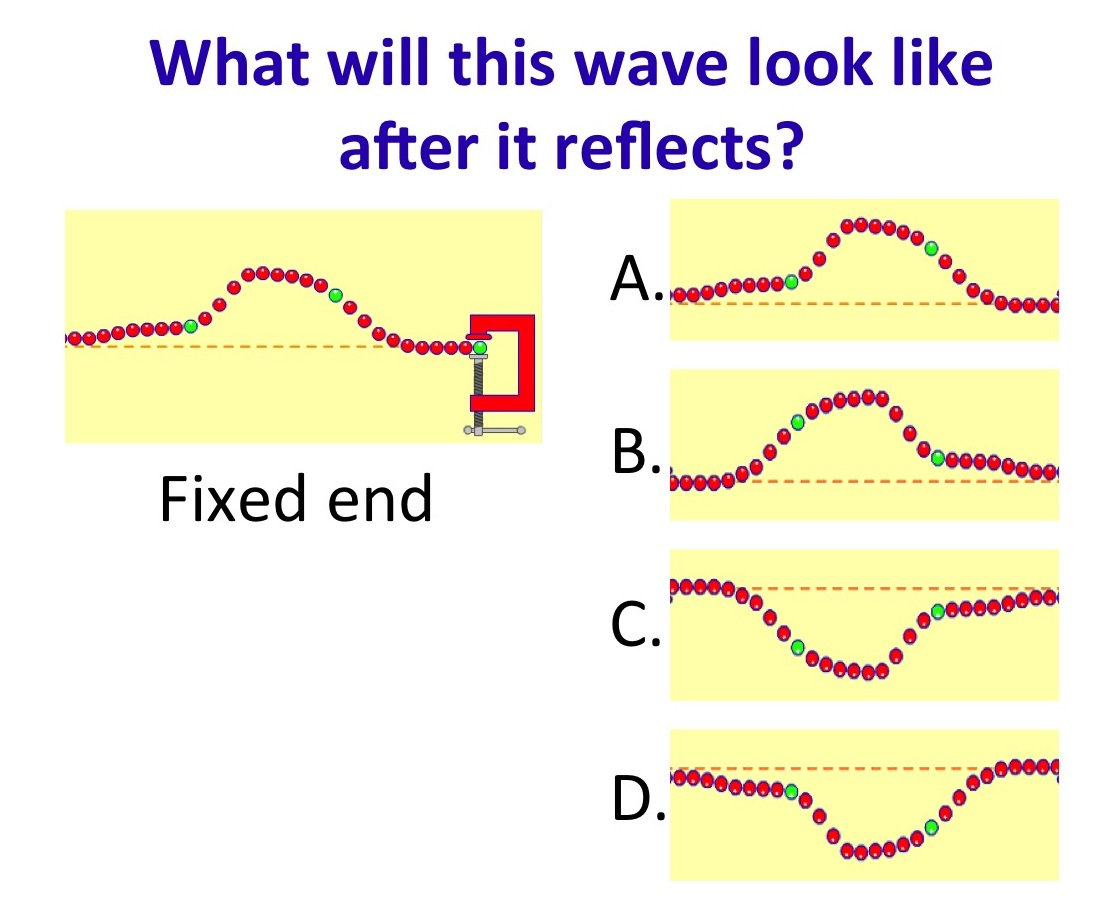Students predict wave behavior in Wave on a String. Answer = C.

Or, you might ask students to predict behavior quantitatively. With the Circuit Construction Kit, you can ask students to predict the brightness of a bulb, magnitude of current, or other variables.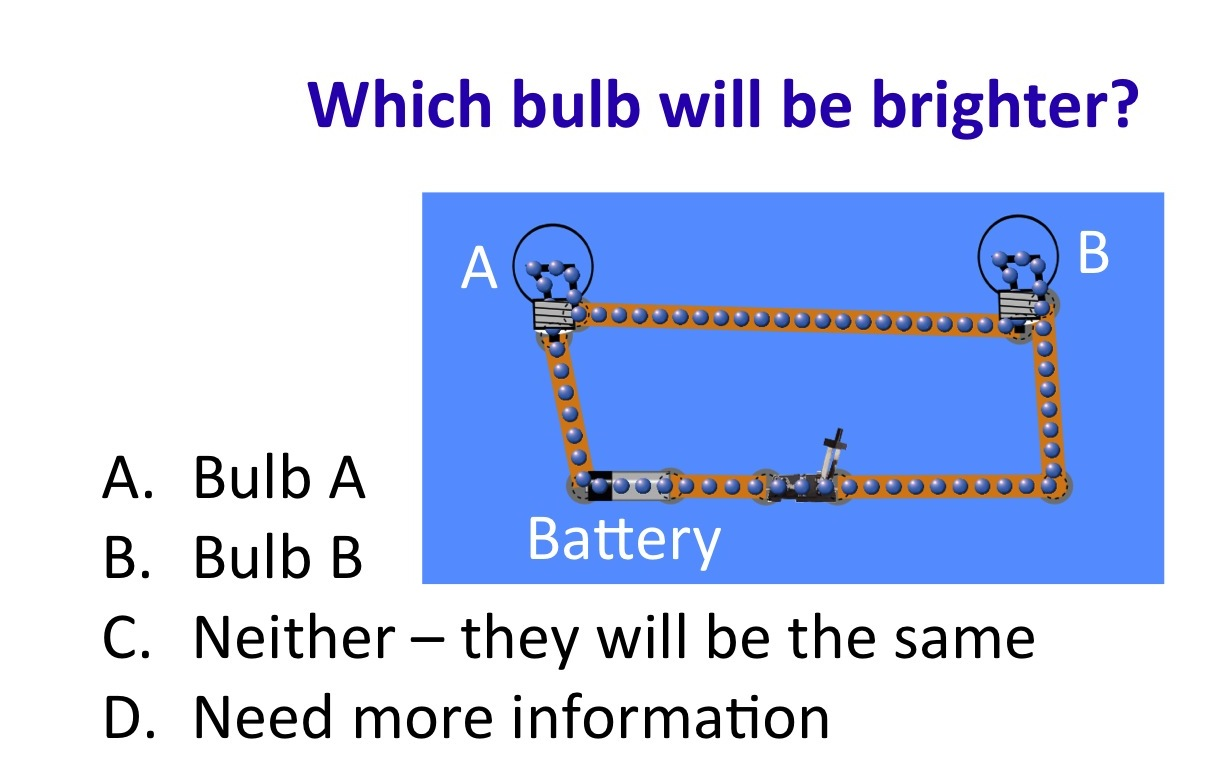Students predict bulb brightness in Circuit Construction Kit. Answer = C.

#### Open-ended prediction

It can be very valuable for students to generate their own answers. This can be done by posing any prediction question and then waiting before showing the answer options. This can also be useful for generating answer choices for a multiple-choice version of the question.  (For a more structured option, see the interactive lecture demonstration (ILD) section below). An introductory example using Faraday’s Electromagnetic Lab is shown below.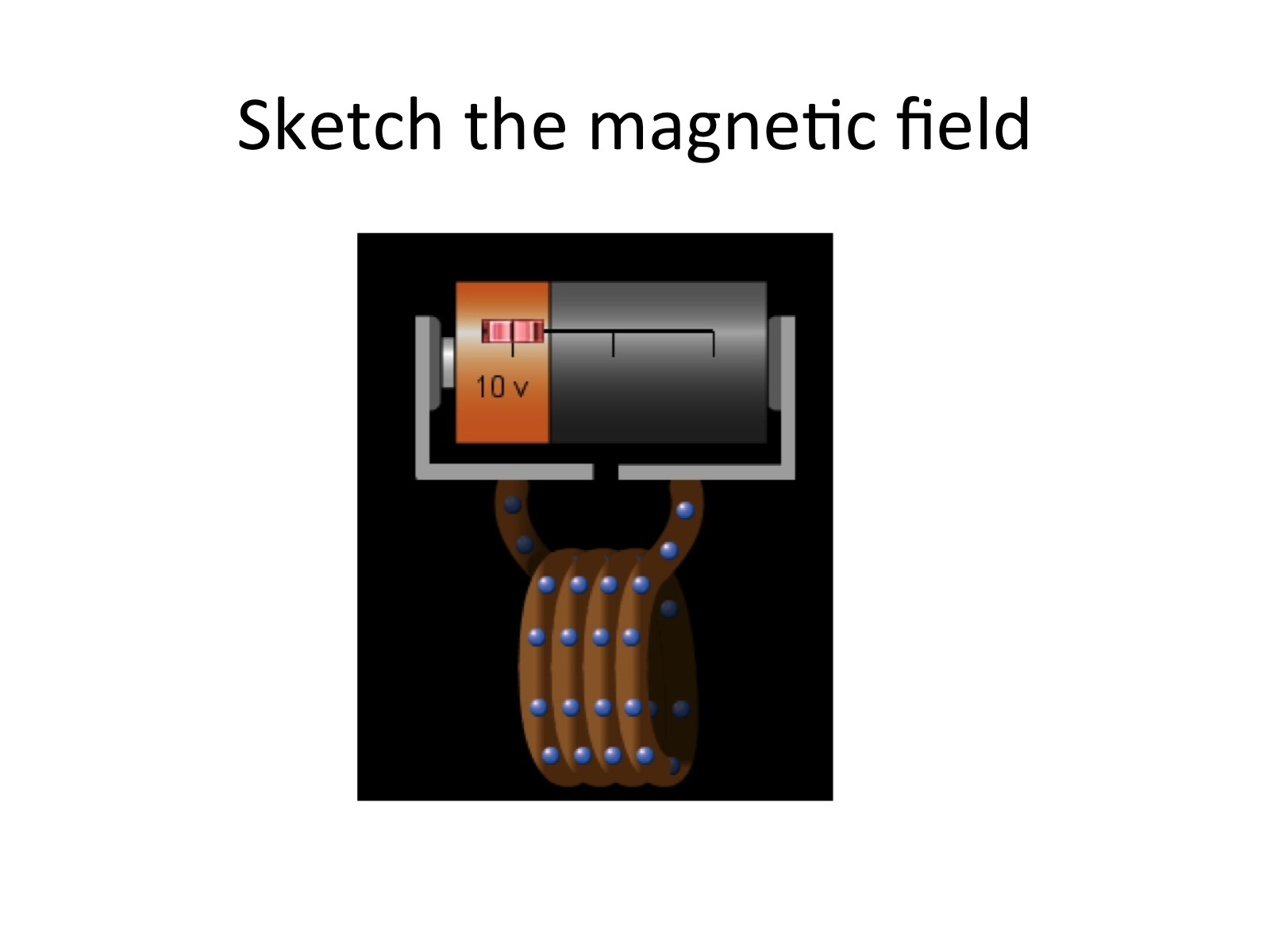An open-ended question with Faraday’s Electromagnetic LabThen, the multiple-choice options are shown. Answer = A.

#### Contrasting Cases

You may ask students to compare or rank different cases in order to highlight key relationships.

For example, in the question below, using the Projectile Motion simulation students need to identify the key variables at play (the ratio of horizontal to vertical components of the initial velocity) in order to rank the following scenarios: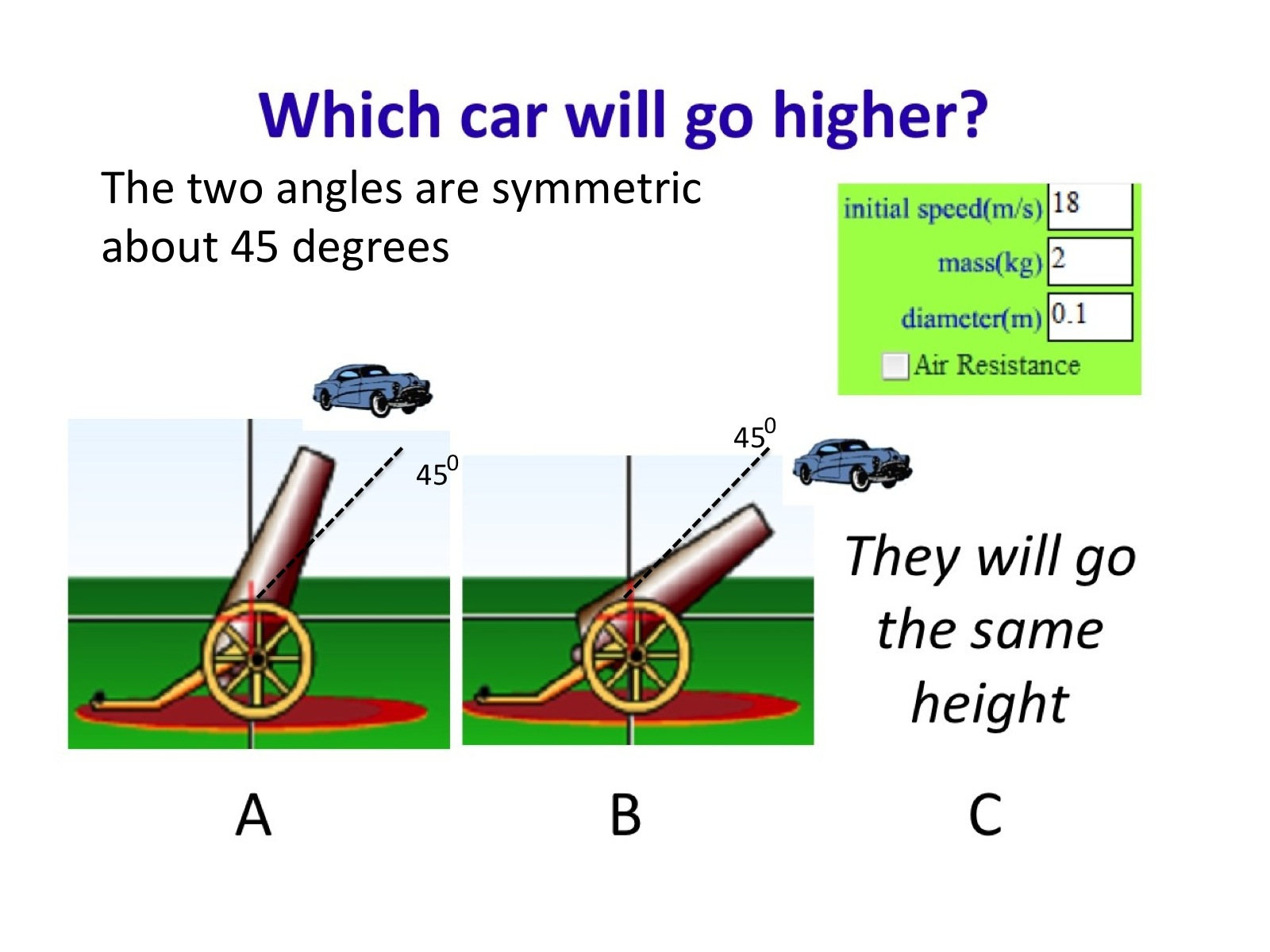Students rank two cases in Projectile Motion; symmetry about 45 degrees results in students being tempted to vote “C” since they often recall that something is maximized at 45 degrees. Answer = A.

Or, in the Buoyancy simulation, comparing the buoyancy requires students to recognize the importance of the amount of the object that is submerged, as well as its' density.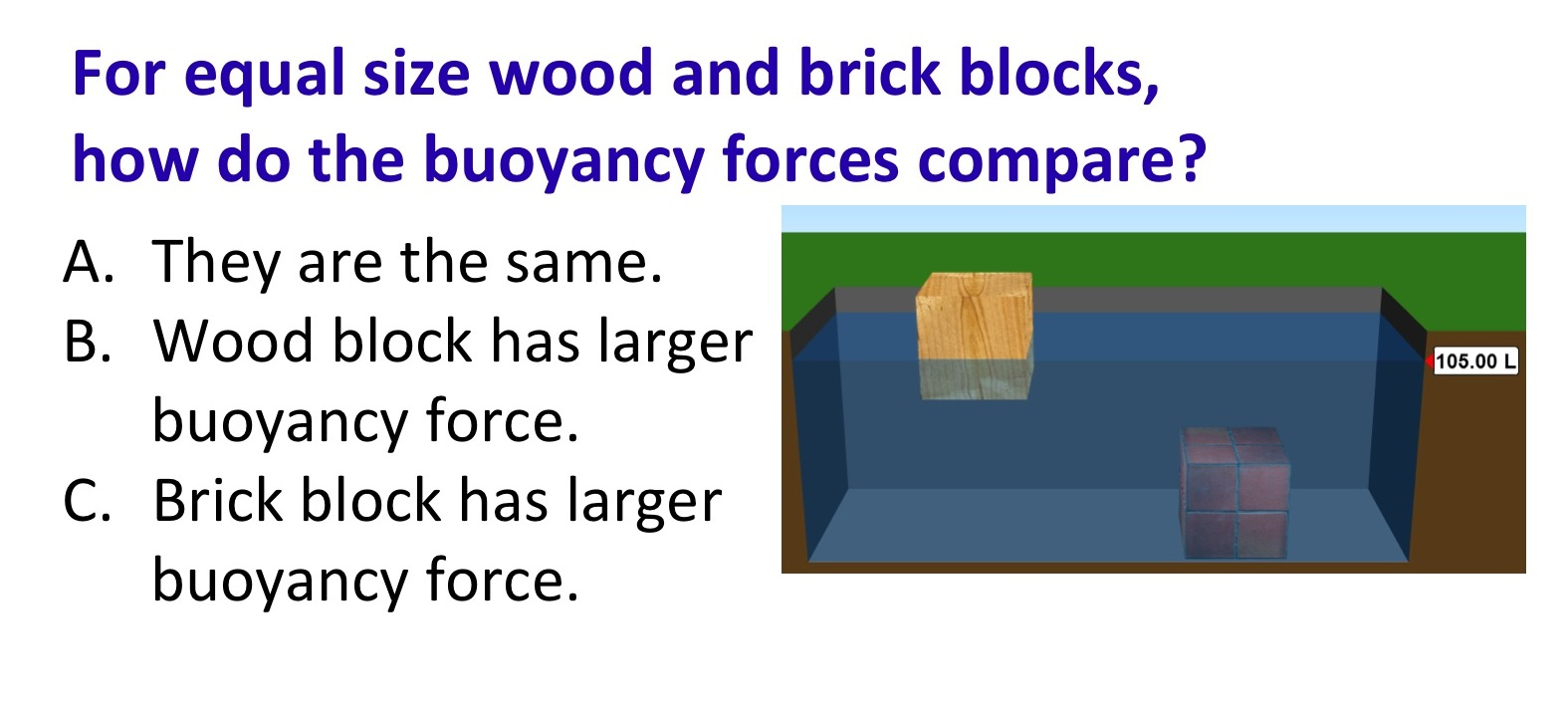Students compare forces in Buoyancy. Answer = C.

#### Interpreting representations

PhET simulations use a variety of representations (graphs, fields, vectors, etc.), and clicker questions can ask students to make sense of these representations.

For example, in the Wave Interference simulation, students may be asked to interpret a common two-dimensional representation of three-dimensional waves.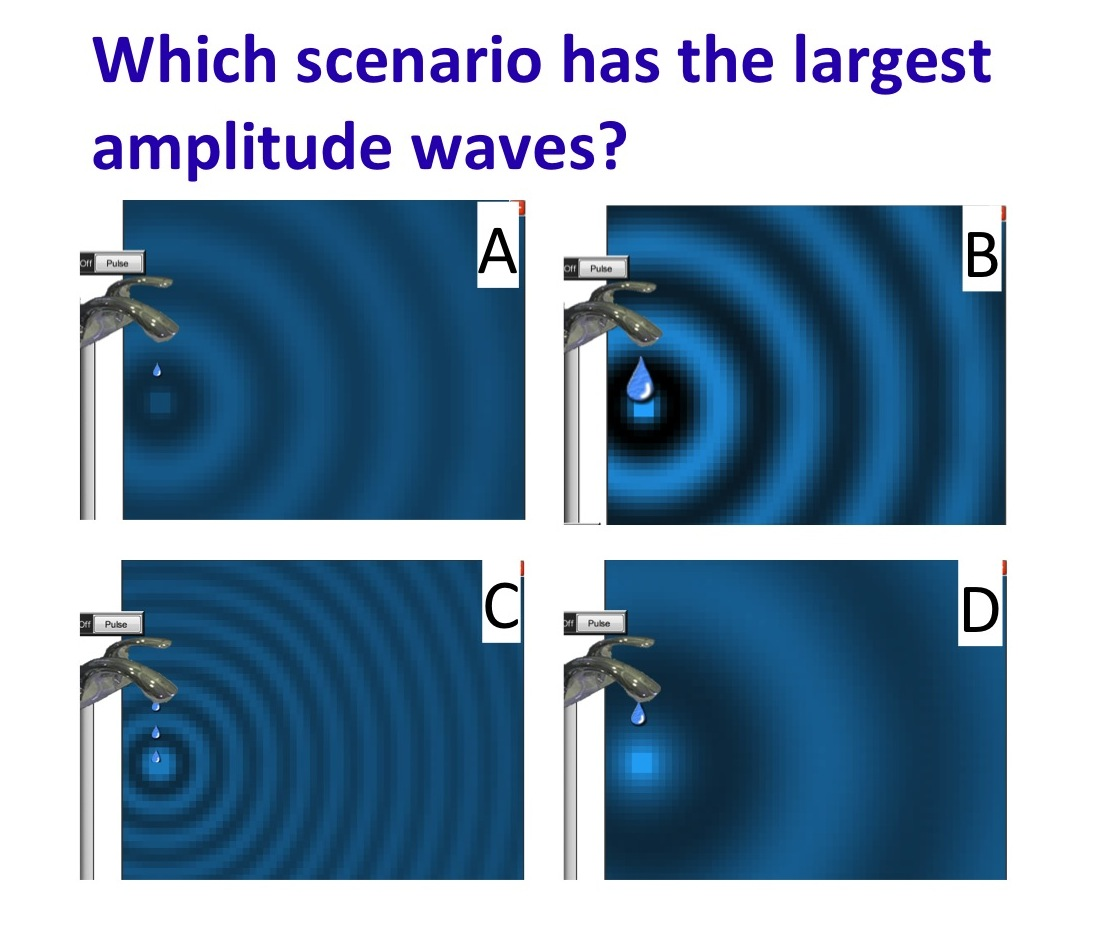Students interpret representations in Wave Interference. Answer = B.

Or, in Energy Skate Park Basics, students are asked to coordinate the two-dimensional representation of the skater's position on an x-y plane, with a histogram representing total, kinetic, and potential energy (Answer = B).### How do I use PhET simulations as an Interactive Lecture Demonstration?

In an interactive lecture demonstration, students predict what will happen in a particular situation, and discuss with their neighbors, before the instructor conducts the experiment and facilitates student discussion about the result. Learning from interactive lecture demonstrations has been shown to improve relative to traditional demonstrations, where students often don't change their ideas about the physics concepts (Sokoloff and Thornton 1997). By first predicting what will happen, students are more interested and motivated to pay attention to, and remember, the result of the experiment.  See the video at right for an in-depth look at this technique.

Steps in an interactive lecture demonstration (ILD):

1. Students predict the outcome of an experiment (writing it down on their own)
2. Discuss with their neighbors, during which they can use the simulation as a common experience or visual upon which to build their discussion
3. Discuss predictions as a whole class, perhaps using a clicker question
4. Observe the simulation, as led by the instructor
5. Discuss the outcome as a whole class

To use PhET as an ILD, you can use the PhET simulations as virtual experiments, where students are asked to predict what will happen in the simulation as different parameters are changed.

For example, the Buoyancy simulation has a “playground” tab where you can modify the mass, density, and volume of the brick, as well as the fluid in which it is immersed. Some questions you might pose:

• Will a wood block sink or float when placed in olive oil (density 0.92 kg/L)
• For a 10 kg brick block immersed in water, what will its’ apparent weight be?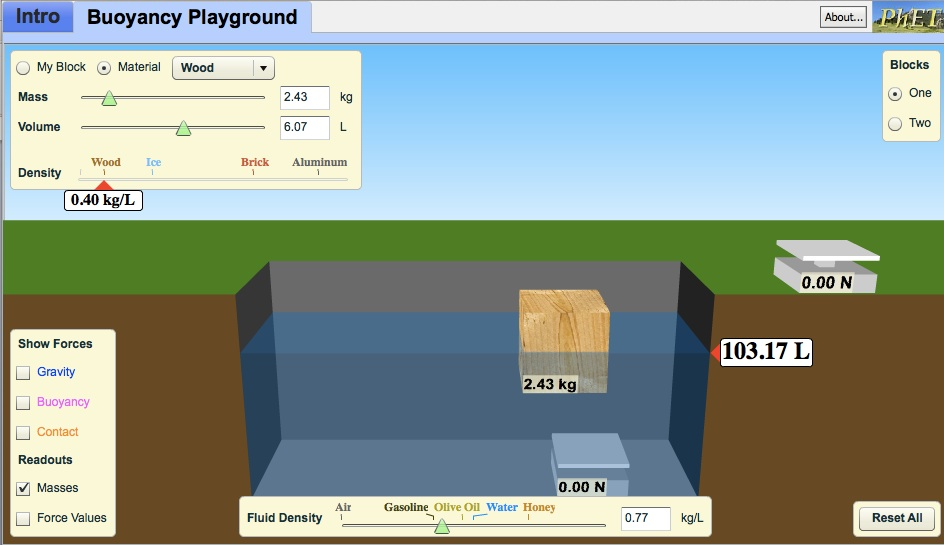A block with customizable properties in the Buoyancy simulation

Some ILDs ask students to sketch or graph their predictions. For example, using the Moving Man simulation, you can have students sketch their predictions for the position versus time graph in a particular scenario, first on their own, and then discussing with their neighbors: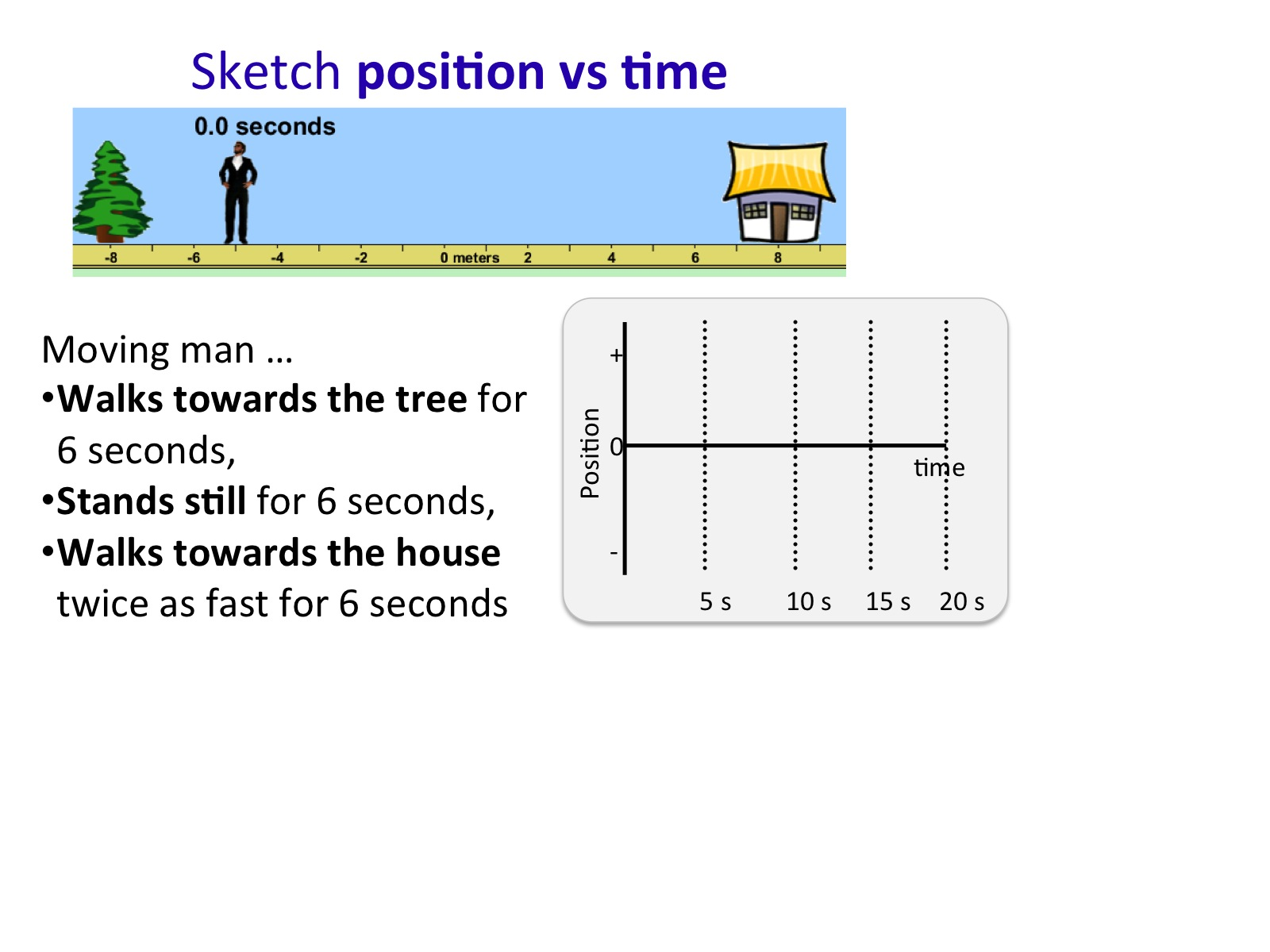Students are given a task using Moving Man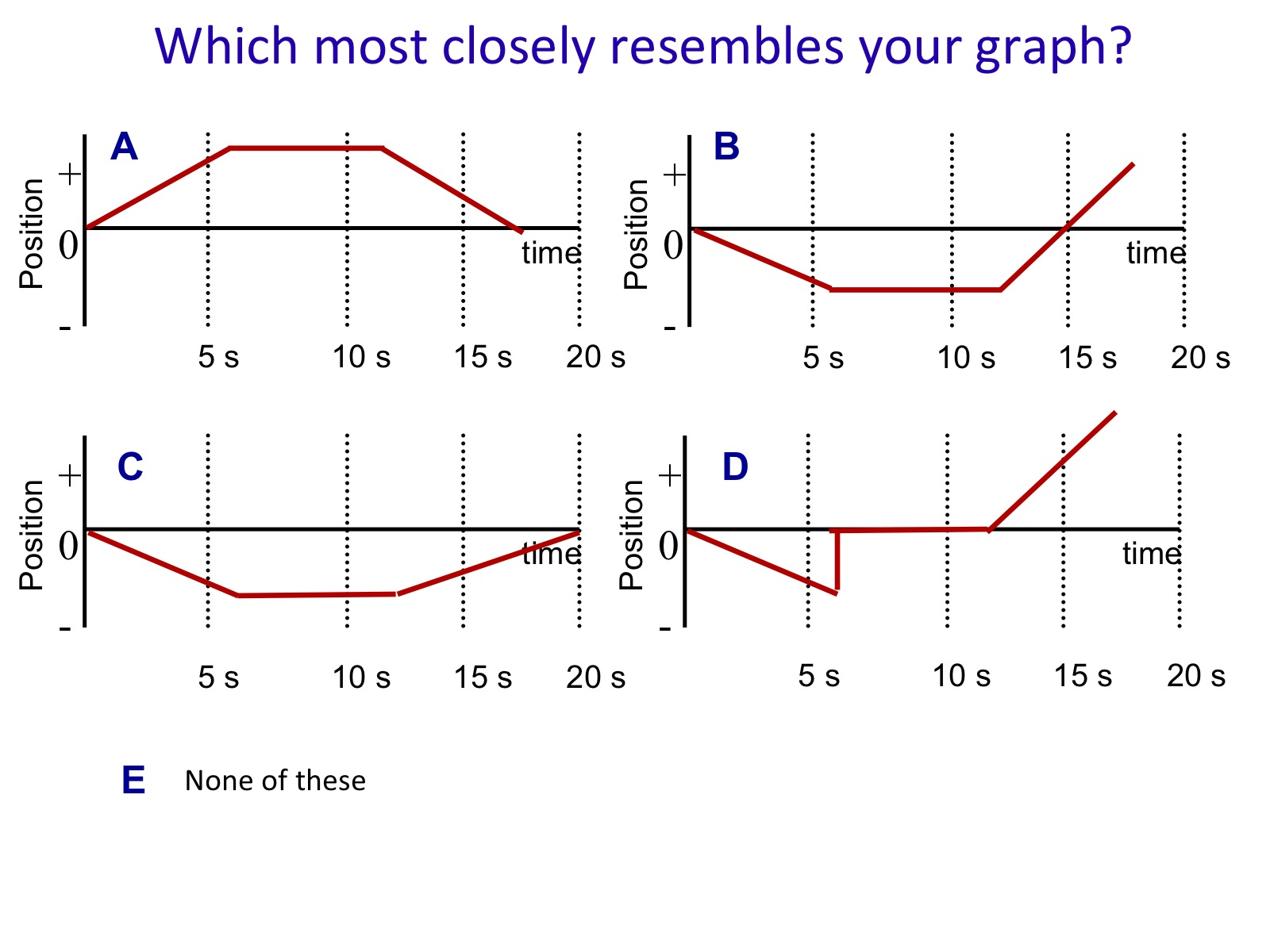Students vote on different options. Answer = B.

The most common choices can then be used to turn the question into a closed, multiple-choice question, so you can conduct a vote.

Then the instructor can conduct the demonstration with the simulation, and hold a whole-class discussion about the results. The discussion can be extended by choosing to show the velocity vector along with the graph, to help make the connection between movement in the x-direction, and the graph of velocity versus time.

### References

#### Related Teaching Method

PhET Interactive Simulations

#### Related Workshop

PhET Interactive Simulations